Next: Two-Body Elastic Collisions Up: Collisions Previous: Introduction

# Collision Operator

Plasma physics can be regarded formally as a closure of Maxwell's equations by means of constitutive relations: that is, expressions specifying the charge density,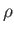, and the current density,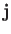, in terms of the electric and magnetic fields,and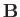(Hazeltine and Waelbroeck 2004). Such relations can be expressed in terms of the microscopic distribution functions,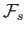, for each plasma species: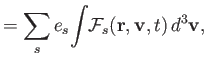(3.1)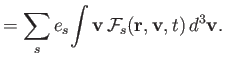(3.2)

Here,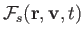is the exact microscopic phase-space density of plasma species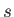(with chargeand mass) near point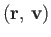at time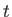(Reif 1965). The distribution functionis normalized such that its velocity integral is equal to the particle number density in coordinate space. In other words,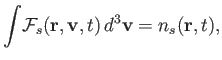(3.3)

where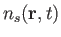is the number (per unit volume) of species-particles near point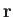at time.

If we could determine eachin terms of the electromagnetic fields then Equations (3.1) and (3.2) would give us the desired constitutive relations. In fact, the time evolution of the various distribution functions is determined by particle conservation in phase-space, which requires that (Reif 1965)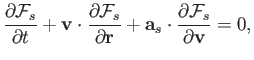(3.4)

where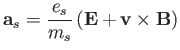(3.5)

is the species-particle acceleration under the influence of theandfields.

Equation (3.4) is easy to derive because it is exact, taking into account all lengthscales from the microscopic to the macroscopic. Note, in particular, that there is no statistical averaging involved in Equation (3.4). It follows that the microscopic distribution function,, is essentially a sum of Dirac delta-functions, each following the detailed trajectory of a single particle. Consequently, the electromagnetic fields appearing in Equation (3.4) are extremely spiky on microscopic scales. In fact, solving Equation (3.4) is equivalent to solving the classical electromagnetic many-body problem, which is a completely hopeless task.

A much more useful equation can be extracted from Equation (3.4) by ensemble averaging (Reif 1965). The average distribution function,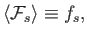(3.6)

is smooth on microscopic lengthscales, and is closely related to actual experimental measurements. Here, angle brackets denote an ensemble average. Similarly, the ensemble-averaged electromagnetic fields are also smooth. Unfortunately, the extraction of an ensemble-averaged equation from Equation (3.4) is mathematically challenging, and invariably involves some level of approximation. The problem is that, because the exact electromagnetic fields depend on particle trajectories,andare not statistically independent of. In other words, as a consequence of correlations between the distribution function and the electromagnetic fields on microscopic lengthscales, the ensemble average of the nonlinear acceleration term in Equation (3.4) is such that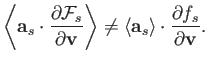(3.7)

It is convenient to write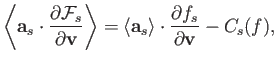(3.8)

where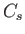is an operator that accounts for the correlations. Because the most important correlations result from close encounters between particles,is known as the collision operator (for species). It is not generally a linear operator, and usually involves the distribution functions of both colliding species (the subscript in the argument ofis omitted for this reason). Hence, the ensemble-averaged version of Equation (3.4) is written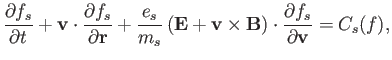(3.9)

whereandare now understood to be the smooth, ensemble-averaged electromagnetic fields. Of course, in a weakly coupled plasma, the dominant collisions are two-particle Coulomb collisions. Equation (3.9) is generally known as the kinetic equation.Next: Two-Body Elastic Collisions Up: Collisions Previous: Introduction
Richard Fitzpatrick 2016-01-23